Next: D.2 Bulge Profile Up: D. Galaxy Surface Brightness Previous: D. Galaxy Surface Brightness   Contents

# D.1 Sersic Law

The properties of galaxy surface brightness radial profiles can be derived in a general form using Sersic law, first introduced by [Sersic 1968] and also known as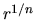law or generalized de Vaucouleurs law. This can be written as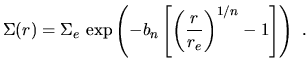(D.1)

where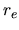is the effective radius, or the radius within which the galaxy emits half its brightness,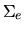is the surface brightness atand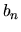is a positive parameter that, for a given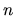, can be determined from the definition ofand. The value ofdetermines the degree of concentration of the profile, quantified e.g. by the fraction of energy emitted within a given number of effective radii, the profile being steeper or less concentrated for higherand conversely flatter or less concentrated for lower. Particularly interesting special cases are the bulge-like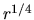profile for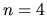and the disk-like exponential profile for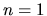, which will be discussed in greater detail in Sections D.2 and D.3.

According to Equation D.1, the brightness integrated within a given radius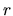is given by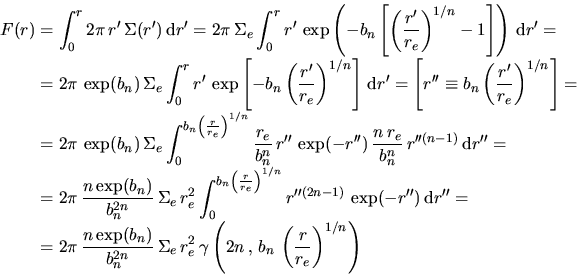(D.2)

where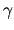is the incomplete gamma function. The total brightness predicted by the profile is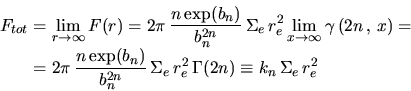(D.3)

where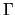is the gamma function. This relation, remembering that, by definition of effective radius, it is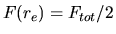, can be used to obtain an equation linkingand. After cancellation of common terms, one obtains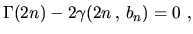(D.4)

a non-linear equation which can only be solved numerically, e.g. via the Newton Method (see Section 9.7 in [Press et al. 1996]). Values ofand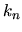corresponding to integer values offrom 1 to 10 are given in Table D.1.1 1 . 6783470 11.948495 2 3 . 6720608 16.310881 3 5 . 6701554 19.743758 4 7 . 6692495 22.665234 5 9 . 6687149 25.251949 6 11 . 668363 27.597728 7 13 . 667757 29.759676 8 15 . 667704 31.774676 9 17 . 667636 33.669429 10 19 . 667567 35.463170Next: D.2 Bulge Profile Up: D. Galaxy Surface Brightness Previous: D. Galaxy Surface Brightness   Contents
Mattia Vaccari 2000-12-05